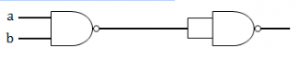## Combinational-Circuits

 Question 1

In the following truth table, V = 1 if and only if the input is valid.What function does the truth table represent?

 A Priority encoder B Decoder C Multiplexer D Demultiplexer
Digital-Logic-Design       Combinational-Circuits       GATE 2013
Question 1 Explanation:
It is a 22 × 2 encoder. The inputs have priorities. So, it is a priority encoder.
 Question 2

The amount of ROM needed to implement a 4 bit multiplier is

 A 64 bits B 128 bits C 1 Kbits D 2 Kbits
Digital-Logic-Design       Combinational-Circuits       GATE 2012
Question 2 Explanation:
To implement a 4-bit multiplier we need to store all the possible combinations of 24 x 24 inputs and their corresponding 8 output bits. The total ROM size needed = 28 x 8 bits = 211 bits = 2 Kbits.
Hence option D is the answer.
 Question 3

A circuit outputs a digit in the form of 4 bits. 0 is represented by 0000, 1 by 0001, ..., 9 by 1001. A combinational circuit is to be designed which takes these 4 bits as input and outputs 1 if the digit ≥ 5, and 0 otherwise. If only AND, OR and NOT gates may be used, what is the minimum number of gates required?

 A 2 B 3 C 4 D 5
Digital-Logic-Design       Combinational-Circuits       GATE 2004
Question 3 Explanation:= A + BD + BC
= A + B (D + C)
So minimum two OR gates and 1 AND gate is required. Hence, in total minimum 3 gates is required.
 Question 4
The combinational circuit given below is implemented with two NAND gates. To which of the following individual gates is its equivalent?A NOT B OR C AND D XOR
Digital-Logic-Design       Combinational-Circuits       Nielit Scientist-B CS 22-07-2017
Question 4 Explanation:
[(a.b)'. (a.b)' ]'= ((a.b)')' + ((a.b)')'
=(a.b)+(a.b)
=(a.b)
There are 4 questions to complete.

Register Now